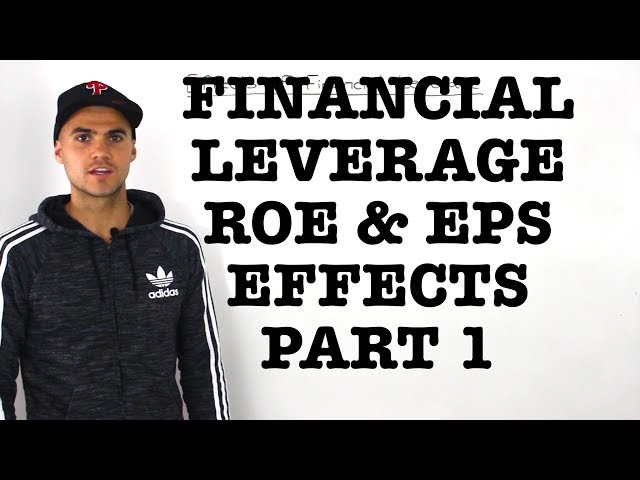# Financial Leverage Effects on EPS and ROE - Ryerson UniversityTLDR Financial leverage, through the use of debt, can impact earnings per share and return on equity, with changes in economic conditions leading to fluctuations in these metrics.

## Key insights

• 💰
The firm's earnings before interest and taxes can be significantly affected by economic conditions, with a 30% increase in an expansion and a 40% decrease in a recession.
• 💰
The effects of financial leverage on return on equity and earnings per share can be analyzed by comparing the measures before and after introducing leverage.
• 💵
Understanding the impact of financial leverage on earnings is crucial for evaluating a company's profitability and potential risks.
• 💰
"In a recession, the return on equity is 70.14%, while in an expansion it is 22.29%."
• 💰
The percentage change in return on equity is directly related to the percentage change in earnings before interest and taxes, as the numerator of the ratio drives the overall increase or decrease.
• 💰
The earnings per share can increase or decrease based on the performance of the economy, with a potential 30% increase or 40% decrease.
• 💸
"Let's move on to a scenario where this same firm is gonna pay 30% of taxes and see what changes with the return on equity and earnings per share."
• 💰
The return on equity (ROE) varies significantly depending on the economic scenario, with the recession having the lowest ROE at 7.2%, the normal economy at 12%, and the expansion at 15.6%.

## Q&A

• What is the impact of financial leverage on earnings per share and return on equity?

Financial leverage, or debt, can impact earnings per share and return on equity by causing fluctuations in these metrics.

• How is net income calculated for a company with no debt and no taxes?

The net income of a company with no debt and no taxes is equal to the earnings before interest and taxes.

• What is the difference between an unleveraged and a leveraged company?

An unleveraged company has no debt, while a leveraged company has debt.

• How is the return on equity calculated?

The return on equity is calculated by dividing the net income by the equity amount.

• How does the change in earnings before interest and taxes affect earnings per share and return on equity?

The change in earnings before interest and taxes is reflected in the change in earnings per share and return on equity, with a 30% increase and 40% decrease respectively.

## Timestamped Summary

• 📊
00:00
Financial leverage (debt) can impact earnings per share and return on equity, with this video analyzing two scenarios (with and without taxes) and providing information on shares and their value.
• 💰
03:48
The net income is determined by adjusting the earnings before interest and taxes based on different economic conditions, and then subtracting the interest.
• 📚
06:02
The net income of an unleveraged company is equal to the earnings before interest and taxes, while a leveraged company has debt.
• 📊
08:20
The return on equity for the firm is 10.29%, calculated by dividing net income by equity amount, and the percentage change can be determined by comparing it to the figure in a normal economy.
• 📈
10:20
A 30% increase in return on equity occurs during expansion, while a 40% decrease occurs during a recession, with a decrease in earnings before interest and taxes.
• 💰
12:57
The earnings per share is calculated by dividing net income by the number of shares, and the percentage change in EPS can be determined by subtracting the base amount from the new amount and dividing it by the base amount.
• 💰
16:33
The firm's earnings before taxes are the same as earnings before interest, but the amount of taxes paid differs due to a 30% tax rate, resulting in different net income figures and varying return on equity percentages in different economic scenarios.
• 📚
19:53
The change in earnings before interest and taxes affects earnings per share and return on equity, with a 30% increase and 40% decrease respectively, even after taxes are accounted for.Report the articleThank you for the feedback